## 为什么要用线段树

• 将这个区间上的所有数累加，然后返回
• 先求前n项的前缀和，然后将这期间差相减

## 线段树例子

• 数字之和——总数字之和 = 左区间数字之和 + 右区间数字之和
• 最大公因数(GCD)——总GCD = gcd( 左区间GCD , 右区间GCD );
• 最大值——总最大值=max(左区间最大值，右区间最大值)

• 众数——只知道左右区间的众数，没法求总区间的众数
• 01序列的最长连续零——只知道左右区间的最长连续零，没法知道总的最长连续零

## 线段树的递归模版（区间和模版）

nums[index] += c

### 区间修改

nums[left, right] += c

## 线段树思路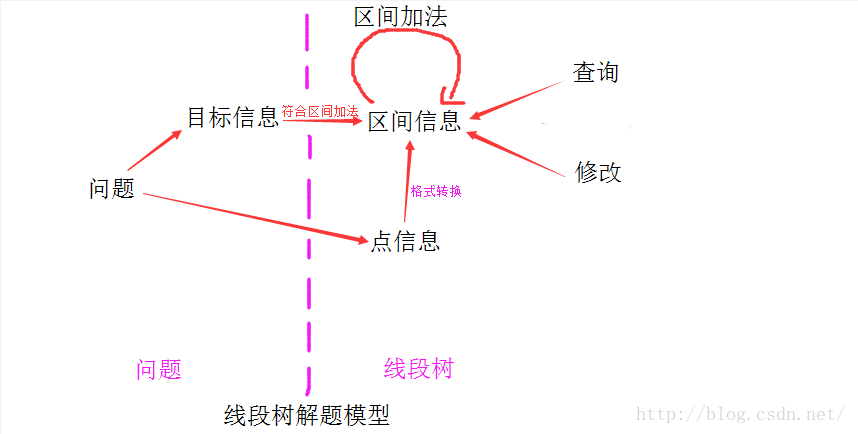## 题目练习

### 最大公因数

update(L,R, c, l, r, root)代表给L到R的所有数都乘以c

## 扫描线

]]>
<blockquote> <p>本文参考了岩之痕的<a href="https://blog.csdn.net/zearot/article/details/52280189" target="_blank" rel="noopener">线段树从零开始</a>和<a href="https://www.cnblogs.com/AC-King/p/7789013.html" target="_blank" rel="noopener">线段树详解</a>，原理可以在原文细看，本文主要是对线段树的几个例题进行实现，以备复习</p> </blockquote> <h2 id="为什么要用线段树"><a href="#为什么要用线段树" class="headerlink" title="为什么要用线段树"></a>为什么要用线段树</h2><p>有这样一个场景，给定一个数组，让求任意区间的累加和。</p> <p>一般有两种算法</p> <ul> <li>将这个区间上的所有数累加，然后返回</li> <li>先求前n项的前缀和，然后将这期间差相减</li> </ul> <p>但是，如果对其中任意一个数据进行操作，就会对累加和的时间复杂度有影响，第一种只需要修改一个元素，第二种却需要将其后的所有前缀和都进行修改。所以第一种查询费时间修改不费时间，第二种查询不费时间修改费时间。线段树就是为了适应修改和统计操作而设计的。</p>
【面经】今日头条部分编程算法题 https://wuzequn.com/2019/03/14/interview-coding-bytedance/ 2019-03-14T14:17:17.000Z 2019-08-12T14:12:47.855Z

• 指定一个元素+1
• 所有的*2

## 自然数排列

0123456791011121314.. 自然数这样顺次排下去，给一个index，找出对应的数字是什么

## 无向图的最小环

]]>
<blockquote> <p>本文收集2018年实验室实习面试部分编程面经以供复习<br></p></blockquote>
Leetcode 周赛模拟 【2】 https://wuzequn.com/2018/10/25/Leetcode-virtual-contest-2/ 2018-10-25T14:07:19.000Z 2019-08-12T14:12:47.847Z

# Weekly Contest 83

## 830. Positions of Large Groups [AC]

Leetcode 830

In a string S of lowercase letters, these letters form consecutive groups of the same character.

For example, a string like S = “abbxxxxzyy” has the groups “a”, “bb”, “xxxx”, “z” and “yy”.

Call a group large if it has 3 or more characters. We would like the starting and ending positions of every large group.

The final answer should be in lexicographic order.

Example 1:

Example 2:

Example 3:

Note: 1 <= S.length <= 1000

## 831. Masking Personal Information [AC]

Leetcode 831

We are given a personal information string S, which may represent either an email address or a phone number.

We would like to mask this personal information according to the following rules:

We define a name to be a string of length ≥ 2 consisting of only lowercase letters a-z or uppercase letters A-Z.

An email address starts with a name, followed by the symbol ‘@’, followed by a name, followed by the dot ‘.’ and followed by a name.

All email addresses are guaranteed to be valid and in the format of “name1@name2.name3“.

To mask an email, all names must be converted to lowercase and all letters between the first and last letter of the first name must be replaced by 5 asterisks ‘*’.

1. Phone number:

A phone number is a string consisting of only the digits 0-9 or the characters from the set {‘+’, ‘-‘, ‘(‘, ‘)’, ‘ ‘}. You may assume a phone number contains 10 to 13 digits.

The last 10 digits make up the local number, while the digits before those make up the country code. Note that the country code is optional. We want to expose only the last 4 digits and mask all other digits.

The local number should be formatted and masked as “--1111”, where 1 represents the exposed digits.

To mask a phone number with country code like “+111 111 111 1111”, we write it in the form “+--*-1111”. The ‘+’ sign and the first ‘-‘ sign before the local number should only exist if there is a country code. For example, a 12 digit phone number mask should start with “+-“.

Note that extraneous characters like “(“, “)”, “ “, as well as extra dashes or plus signs not part of the above formatting scheme should be removed.

Return the correct “mask” of the information provided.

Example 1:

Example 2:

Example 3:

Example 4:

## 829. Consecutive Numbers Sum [AC]

Leetcode 829

Given a positive integer N, how many ways can we write it as a sum of consecutive positive integers?

Example 1:

Example 2:

Example 3:

## 828. Unique Letter String [Unsolved]

Leetcode 828

A character is unique in string S if it occurs exactly once in it.

For example, in string S = “LETTER”, the only unique characters are “L” and “R”.

Let’s define UNIQ(S) as the number of unique characters in string S.

For example, UNIQ(“LETTER”) = 2.

Given a string S with only uppercases, calculate the sum of UNIQ(substring) over all non-empty substrings of S.

If there are two or more equal substrings at different positions in S, we consider them different.

Since the answer can be very large, return the answer modulo 10 ^ 9 + 7.

Example 1:

Example 2:

# Weekly Contest 82

## 824. Goat Latin [AC]

Leetcode 824
A sentence S is given, composed of words separated by spaces. Each word consists of lowercase and uppercase letters only.

We would like to convert the sentence to “Goat Latin” (a made-up language similar to Pig Latin.)

The rules of Goat Latin are as follows:

If a word begins with a vowel (a, e, i, o, or u), append “ma” to the end of the word.
For example, the word ‘apple’ becomes ‘applema’.

If a word begins with a consonant (i.e. not a vowel), remove the first letter and append it to the end, then add “ma”.
For example, the word “goat” becomes “oatgma”.

Add one letter ‘a’ to the end of each word per its word index in the sentence, starting with 1.
For example, the first word gets “a” added to the end, the second word gets “aa” added to the end and so on.
Return the final sentence representing the conversion from S to Goat Latin.

Example 1:

Example 2:

## 825. Friends Of Appropriate Ages [AC]

Leetcode 825

Some people will make friend requests. The list of their ages is given and ages[i] is the age of the ith person.

Person A will NOT friend request person B (B != A) if any of the following conditions are true:

age[B] <= 0.5 * age[A] + 7
age[B] > age[A]
age[B] > 100 && age[A] < 100
Otherwise, A will friend request B.

Note that if A requests B, B does not necessarily request A. Also, people will not friend request themselves.

How many total friend requests are made?

Example 1:

Example 2:

Example 3:

## 826. Most Profit Assigning Work [AC]

Leetcode 826

We have jobs: difficulty[i] is the difficulty of the ith job, and profit[i] is the profit of the ith job.

Now we have some workers. worker[i] is the ability of the ith worker, which means that this worker can only complete a job with difficulty at most worker[i].

Every worker can be assigned at most one job, but one job can be completed multiple times.

# FTRL更新公式和SGD更新公式的等效性

SGD算法的迭代计算公式如下：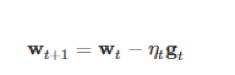FTRL算法的迭代算公式如下：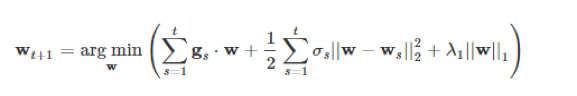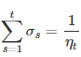λ1是L1正则化系数。在公式2中，arg min算子的内容中由3项组成，如果我们去掉最后面的L1正则化项，公式2就变成下面的公式3：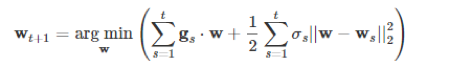（3）式子在换个形式后就等价于梯度下降公式。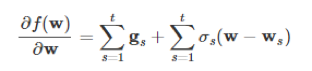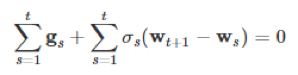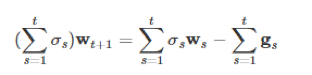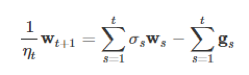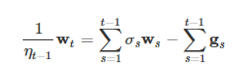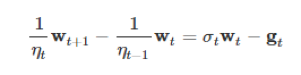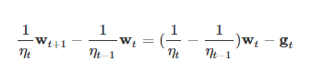]]>
<blockquote> <p>之前的项目中用到了FTRL，在最近的交流中经常被问及这方面的相关基础，发现之前对在线学习的基础知识掌握不全面，以及对OGD和SGD等效性没有理论依据，这里作为总结。</p> </blockquote> <blockquote> <p>本文参考资料<
Leetcode 周赛模拟 【1】 https://wuzequn.com/2018/09/16/Leetcode-virtual-contest-20180910/ 2018-09-16T15:56:59.000Z 2019-08-12T14:12:47.847Z

# Weekly Contest 85

## 836. Rectangle Overlap [AC]

Leetcode 836

A rectangle is represented as a list [x1, y1, x2, y2], where (x1, y1) are the coordinates of its bottom-left corner, and (x2, y2) are the coordinates of its top-right corner.

Two rectangles overlap if the area of their intersection is positive. To be clear, two rectangles that only touch at the corner or edges do not overlap.

Given two (axis-aligned) rectangles, return whether they overlap.

Example 1:

Example 2:

## 838. Push Dominoes [AC]

Leetcode 838

There are N dominoes in a line, and we place each domino vertically upright.

In the beginning, we simultaneously push some of the dominoes either to the left or to the right.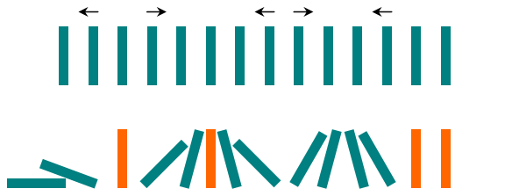After each second, each domino that is falling to the left pushes the adjacent domino on the left.

Similarly, the dominoes falling to the right push their adjacent dominoes standing on the right.

When a vertical domino has dominoes falling on it from both sides, it stays still due to the balance of the forces.

For the purposes of this question, we will consider that a falling domino expends no additional force to a falling or already fallen domino.

Given a string “S” representing the initial state. S[i] = ‘L’, if the i-th domino has been pushed to the left; S[i] = ‘R’, if the i-th domino has been pushed to the right; S[i] = ‘.’, if the i-th domino has not been pushed.

Return a string representing the final state.

Example 1:

Example 2:

## 837. New 21 Game [unsolved]

Leetcode 837

Alice plays the following game, loosely based on the card game “21”.

Alice starts with 0 points, and draws numbers while she has less than K points. During each draw, she gains an integer number of points randomly from the range [1, W], where W is an integer. Each draw is independent and the outcomes have equal probabilities.

Alice stops drawing numbers when she gets K or more points. What is the probability that she has N or less points?

Example 1:

Example 2:

Example 3:

## 839. Similar String Groups [AC]

Leetcode 839

Two strings X and Y are similar if we can swap two letters (in different positions) of X, so that it equals Y.

For example, “tars” and “rats” are similar (swapping at positions 0 and 2), and “rats” and “arts” are similar, but “star” is not similar to “tars”, “rats”, or “arts”.

Together, these form two connected groups by similarity: {“tars”, “rats”, “arts”} and {“star”}. Notice that “tars” and “arts” are in the same group even though they are not similar. Formally, each group is such that a word is in the group if and only if it is similar to at least one other word in the group.

We are given a list A of strings. Every string in A is an anagram of every other string in A. How many groups are there?

Example 1:

# Weekly Contest 84

## 832. Flipping an Image [AC]

Leetcode 832

Given a binary matrix A, we want to flip the image horizontally, then invert it, and return the resulting image.

To flip an image horizontally means that each row of the image is reversed. For example, flipping [1, 1, 0] horizontally results in [0, 1, 1].

To invert an image means that each 0 is replaced by 1, and each 1 is replaced by 0. For example, inverting [0, 1, 1] results in [1, 0, 0].

Example 1:

Example 2:

## 833. Find And Replace in String [AC]

Leetcode 833

To some string S, we will perform some replacement operations that replace groups of letters with new ones (not necessarily the same size).

Each replacement operation has 3 parameters: a starting index i, a source word x and a target word y. The rule is that if x starts at position i in the original string S, then we will replace that occurrence of x with y. If not, we do nothing.

For example, if we have S = “abcd” and we have some replacement operation i = 2, x = “cd”, y = “ffff”, then because “cd” starts at position 2 in the original string S, we will replace it with “ffff”.

Using another example on S = “abcd”, if we have both the replacement operation i = 0, x = “ab”, y = “eee”, as well as another replacement operation i = 2, x = “ec”, y = “ffff”, this second operation does nothing because in the original string S = ‘c’, which doesn’t match x = ‘e’.

All these operations occur simultaneously. It’s guaranteed that there won’t be any overlap in replacement: for example, S = “abc”, indexes = [0, 1], sources = [“ab”,”bc”] is not a valid test case.

Example 1:

Example 2:

## 835. Image Overlap [AC]

Leetcode 835

Two images A and B are given, represented as binary, square matrices of the same size. (A binary matrix has only 0s and 1s as values.)

We translate one image however we choose (sliding it left, right, up, or down any number of units), and place it on top of the other image. After, the overlap of this translation is the number of positions that have a 1 in both images.

(Note also that a translation does not include any kind of rotation.)

What is the largest possible overlap?

Example 1:

## 834. Sum of Distances in Tree [TLE]

Leetcode 834

An undirected, connected tree with N nodes labelled 0…N-1 and N-1 edges are given.

The ith edge connects nodes edges[i] and edges[i] together.

Return a list ans, where ans[i] is the sum of the distances between node i and all other nodes.

Example 1:

]]>
【总结】零星算法模板总结 https://wuzequn.com/2018/09/08/leetcode-summary-some-sample/ 2018-09-08T05:01:13.000Z 2019-08-12T14:12:47.866Z

# 水塘抽样

Leetcode 382

Given a singly linked list, return a random node’s value from the linked list. Each node must have the same probability of being chosen.

What if the linked list is extremely large and its length is unknown to you? Could you solve this efficiently without using extra space?

Example:

## Random Pick Index

Leetcode 398

Given an array of integers with possible duplicates, randomly output the index of a given target number. You can assume that the given target number must exist in the array.

Note:
The array size can be very large. Solution that uses too much extra space will not pass the judge.

Example:

# 线段树

## Count of Smaller Numbers After Self

Leetcode 315

You are given an integer array nums and you have to return a new counts array. The counts array has the property where counts[i] is the number of smaller elements to the right of nums[i].

Example:

# 子串和满足条件的最短长度

## Minimum Size Subarray Sum

Leetcode 209

Given an array of n positive integers and a positive integer s, find the minimal length of a contiguous subarray of which the sum ≥ s. If there isn’t one, return 0 instead.

Example:

## 上一题数组中包含正负，找和为target的最小长度

Leetcode 862

Return the length of the shortest, non-empty, contiguous subarray of A with sum at least K.

If there is no non-empty subarray with sum at least K, return -1.

Example 1:

Example 2:

Example 3:

# 快排相关

## 快排优化

• 基准随机化算法

• 将前后中间三个数取中值作为标兵元素

• 在数组大小等于一定范围的时候，改为插入排序，防止排序退化
• 将相等的数字聚集到一起，然后跳过此处的排序

# 牛顿法

Leetcode 69

Implement int sqrt(int x).

Compute and return the square root of x, where x is guaranteed to be a non-negative integer.

Since the return type is an integer, the decimal digits are truncated and only the integer part of the result is returned.

Example 1:

Example 2:

# 快速幂

## Pow(x, n)

Leetcode 50

Implement pow(x, n), which calculates x raised to the power n (xn).

# 全排列相关

Leetcode 60

## 字母全排列

1. 从最后向前找相邻的两个元素是的i < ii
2. 然后再从最后向前找一个元素使得i < j
3. i和j交换，然后将ii之后的元素reverse排序

nextPermutation的头文件是algorithm

# 面试题集合

## 面试题5

M=
| |A |B |C |
|—-|:–:|:–:|:–:|
|A |B,C |C |A |
|B |A,C |C |C |
|C |A |A |A,B |

S={A,B,C}

]]>
<blockquote> <p>这里整理一些零星的算法，作为记录…<br></p></blockquote>
Leetcode DP及贪心算法总结 https://wuzequn.com/2018/09/05/leetcode-summary-dp-and-greedy/ 2018-09-04T16:39:56.000Z 2019-08-12T14:12:47.862Z

# 贪心

## Jump Game

Leetcode 55

Given an array of non-negative integers, you are initially positioned at the first index of the array.

Each element in the array represents your maximum jump length at that position.

Determine if you are able to reach the last index.

Example 1:

Example 2:

## Majority Element II

Leetcode 229

Given an integer array of size n, find all elements that appear more than ⌊ n/3 ⌋ times.

Note: The algorithm should run in linear time and in O(1) space.

Example 1:

Example 2:

## Queue Reconstruction by Height

Leetcode 406

Suppose you have a random list of people standing in a queue. Each person is described by a pair of integers (h, k), where h is the height of the person and k is the number of people in front of this person who have a height greater than or equal to h. Write an algorithm to reconstruct the queue.

Note:
The number of people is less than 1,100.

Example

## Minimum Number of Arrows to Burst Balloons

Leetcode 452

There are a number of spherical balloons spread in two-dimensional space. For each balloon, provided input is the start and end coordinates of the horizontal diameter. Since it’s horizontal, y-coordinates don’t matter and hence the x-coordinates of start and end of the diameter suffice. Start is always smaller than end. There will be at most 104 balloons.

An arrow can be shot up exactly vertically from different points along the x-axis. A balloon with xstart and xend bursts by an arrow shot at x if xstart ≤ x ≤ xend. There is no limit to the number of arrows that can be shot. An arrow once shot keeps travelling up infinitely. The problem is to find the minimum number of arrows that must be shot to burst all balloons.

Example:

## Remove K Digits

Leetcode 402

Given a non-negative integer num represented as a string, remove k digits from the number so that the new number is the smallest possible.

Note:

• The length of num is less than 10002 and will be ≥ k.
• The given num does not contain any leading zero.

Example 1:

Example 2:

Example 3:

# 动态规划

## Gas Station

Leetcode 134

There are N gas stations along a circular route, where the amount of gas at station i is gas[i].

You have a car with an unlimited gas tank and it costs cost[i] of gas to travel from station i to its next station (i+1). You begin the journey with an empty tank at one of the gas stations.

Return the starting gas station’s index if you can travel around the circuit once in the clockwise direction, otherwise return -1.

Note:

• If there exists a solution, it is guaranteed to be unique.
• Both input arrays are non-empty and have the same length.
• Each element in the input arrays is a non-negative integer.

Example 1:

Example 2:

## Counting Bits

Leetcode 338

Given a non negative integer number num. For every numbers i in the range 0 ≤ i ≤ num calculate the number of 1’s in their binary representation and return them as an array.

Example 1:

Example 2:

## House Robber II

Leetcode 213

You are a professional robber planning to rob houses along a street. Each house has a certain amount of money stashed. All houses at this place are arranged in a circle. That means the first house is the neighbor of the last one. Meanwhile, adjacent houses have security system connected and it will automatically contact the police if two adjacent houses were broken into on the same night.

Given a list of non-negative integers representing the amount of money of each house, determine the maximum amount of money you can rob tonight without alerting the police.

Example 1:

Example 2:

## Largest Divisible Subset

Leetcode 368

Given a set of distinct positive integers, find the largest subset such that every pair (Si, Sj) of elements in this subset satisfies:

Si % Sj = 0 or Sj % Si = 0.

If there are multiple solutions, return any subset is fine.

Example 1:

Example 2:

## Best Time to Buy and Sell Stock with Cooldown

Leetcode 309

Say you have an array for which the ith element is the price of a given stock on day i.

Design an algorithm to find the maximum profit. You may complete as many transactions as you like (ie, buy one and sell one share of the stock multiple times) with the following restrictions:

You may not engage in multiple transactions at the same time (ie, you must sell the stock before you buy again).
After you sell your stock, you cannot buy stock on next day. (ie, cooldown 1 day)

Example:

## Word Break

Leetcode 139

Given a non-empty string s and a dictionary wordDict containing a list of non-empty words, determine if s can be segmented into a space-separated sequence of one or more dictionary words.

Note:

• The same word in the dictionary may be reused multiple times in the segmentation.
• You may assume the dictionary does not contain duplicate words.

Example 1:

Example 2:

Example 3:

## Wiggle Subsequence

Leetcode 376

A sequence of numbers is called a wiggle sequence if the differences between successive numbers strictly alternate between positive and negative. The first difference (if one exists) may be either positive or negative. A sequence with fewer than two elements is trivially a wiggle sequence.

For example, [1,7,4,9,2,5] is a wiggle sequence because the differences (6,-3,5,-7,3) are alternately positive and negative. In contrast, [1,4,7,2,5] and [1,7,4,5,5] are not wiggle sequences, the first because its first two differences are positive and the second because its last difference is zero.

Given a sequence of integers, return the length of the longest subsequence that is a wiggle sequence. A subsequence is obtained by deleting some number of elements (eventually, also zero) from the original sequence, leaving the remaining elements in their original order.

Examples:

## Arithmetic Slices

Leetcode 413

A sequence of number is called arithmetic if it consists of at least three elements and if the difference between any two consecutive elements is the same.

Example:

## Regular Expression Matching

Leetcode 10

Given an input string (s) and a pattern (p), implement regular expression matching with support for ‘.’ and ‘*’.

Note:

• s could be empty and contains only lowercase letters a-z.
• p could be empty and contains only lowercase letters a-z, and characters like . or *.

Example 1:

Example 2:

Example 3:

Example 4:

Example 5:

## Wildcard Matching

Leetcode 44

Given an input string (s) and a pattern (p), implement wildcard pattern matching with support for ‘?’ and ‘*’.

‘?’ Matches any single character.
‘*’ Matches any sequence of characters (including the empty sequence).
The matching should cover the entire input string (not partial).

Note:

s could be empty and contains only lowercase letters a-z.
p could be empty and contains only lowercase letters a-z, and characters like ? or *.

Example 1:

Example 2:

Example 3:

Example 4:

Example 5:

## Length of Longest Fibonacci Subsequence

Leetcode 873

A sequence X_1, X_2, …, X_n is fibonacci-like if:

• n >= 3
• X_i + X_{i+1} = X_{i+2} for all i + 2 <= n

Given a strictly increasing array A of positive integers forming a sequence, find the length of the longest fibonacci-like subsequence of A. If one does not exist, return 0.

(Recall that a subsequence is derived from another sequence A by deleting any number of elements (including none) from A, without changing the order of the remaining elements. For example, [3, 5, 8] is a subsequence of [3, 4, 5, 6, 7, 8].)

Example 1:

Example 2:

# 背包

## Ones and Zeroes

Leetcode 474

In the computer world, use restricted resource you have to generate maximum benefit is what we always want to pursue.

For now, suppose you are a dominator of m 0s and n 1s respectively. On the other hand, there is an array with strings consisting of only 0s and 1s.

Now your task is to find the maximum number of strings that you can form with given m 0s and n 1s. Each 0 and 1 can be used at most once.

Note:

• The given numbers of 0s and 1s will both not exceed 100
• The size of given string array won’t exceed 600.

Example 1:

Example 2:

## Partition Equal Subset Sum

Leetcode 416

Given a non-empty array containing only positive integers, find if the array can be partitioned into two subsets such that the sum of elements in both subsets is equal.

Note:

• Each of the array element will not exceed 100.
• The array size will not exceed 200.

Example 1:

Example 2:

## 回文子序列个数

]]>
<blockquote> <p>这里总结一些DP类型的题目，因为贪心一定程度可以认为是一维的DP，所以也做总结<br></p></blockquote>
Leetcode 搜索算法相关整理 https://wuzequn.com/2018/09/03/leetcode-summary-search-algorithm/ 2018-09-02T17:34:27.000Z 2019-08-12T14:12:47.864Z

# 快速搜索相关

## Kth Largest Element in an Array

Leetcode 215

Find the kth largest element in an unsorted array. Note that it is the kth largest element in the sorted order, not the kth distinct element.

## Wiggle Sort II

Leetcode 324

Given an unsorted array nums, reorder it such that nums < nums > nums < nums….

Follow Up: Can you do it in O(n) time and/or in-place with O(1) extra space?

# 并查集

## Longest Consecutive Sequence

Leetcode 128

Given an unsorted array of integers, find the length of the longest consecutive elements sequence.

Your algorithm should run in O(n) complexity.

Example:

# BFS

BFS因为占用的空间比较大且一般时间比较长，所以经常用在需要全部数据都要检索的题目上，一般的类型包括：

• 图上任意两点之间的距离
• 图的拓扑排序
• 树与层数相关的题目，例如树形图的直径，寻找根节点等
• 可能还有其他的类型，之后会来补充…

## Course Schedule （拓扑排序）

Leetcode 207

There are a total of n courses you have to take, labeled from 0 to n-1.

Some courses may have prerequisites, for example to take course 0 you have to first take course 1, which is expressed as a pair: [0,1]

Given the total number of courses and a list of prerequisite pairs, is it possible for you to finish all courses?

## Minimum Height Trees

Leetcode 310

For a undirected graph with tree characteristics, we can choose any node as the root. The result graph is then a rooted tree. Among all possible rooted trees, those with minimum height are called minimum height trees (MHTs). Given such a graph, write a function to find all the MHTs and return a list of their root labels.

Format
The graph contains n nodes which are labeled from 0 to n - 1. You will be given the number n and a list of undirected edges (each edge is a pair of labels).

You can assume that no duplicate edges will appear in edges. Since all edges are undirected, [0, 1] is the same as [1, 0] and thus will not appear together in edges.

Example 1 :

Example 2 :

## Reconstruct Itinerary

Leetcode 332

Given a list of airline tickets represented by pairs of departure and arrival airports [from, to], reconstruct the itinerary in order. All of the tickets belong to a man who departs from JFK. Thus, the itinerary must begin with JFK.

Note:

If there are multiple valid itineraries, you should return the itinerary that has the smallest lexical order when read as a single string. For example, the itinerary [“JFK”, “LGA”] has a smaller lexical order than [“JFK”, “LGB”].
All airports are represented by three capital letters (IATA code).
You may assume all tickets form at least one valid itinerary.

Example 1:

Example 2:

## Populating Next Right Pointers in Each Node

Leetcode 116

Given a binary tree

Populate each next pointer to point to its next right node. If there is no next right node, the next pointer should be set to NULL.

Initially, all next pointers are set to NULL.

Note:

You may only use constant extra space.
Recursive approach is fine, implicit stack space does not count as extra space for this problem.
You may assume that it is a perfect binary tree (ie, all leaves are at the same level, and every parent has two children).

Example:

# DFS

## Binary Tree Maximum Path Sum

Leetcode 124

Given a non-empty binary tree, find the maximum path sum.

For this problem, a path is defined as any sequence of nodes from some starting node to any node in the tree along the parent-child connections. The path must contain at least one node and does not need to go through the root.

Example 1:

Example 2:

## Kth Smallest Element in a BST

Leetcode 230

Given a binary search tree, write a function kthSmallest to find the kth smallest element in it.

Note:
You may assume k is always valid, 1 ≤ k ≤ BST’s total elements.

Example 1:

Example 2:

## Generate Parentheses

Leetcode 22

Given n pairs of parentheses, write a function to generate all combinations of well-formed parentheses.

For example, given n = 3, a solution set is:

## House Robber III

Leetcode 337

The thief has found himself a new place for his thievery again. There is only one entrance to this area, called the “root.” Besides the root, each house has one and only one parent house. After a tour, the smart thief realized that “all houses in this place forms a binary tree”. It will automatically contact the police if two directly-linked houses were broken into on the same night.

Determine the maximum amount of money the thief can rob tonight without alerting the police.

Example 1:

Example 2:

## Decode String

Leetcode 394

Given an encoded string, return it’s decoded string.

The encoding rule is: k[encoded_string], where the encoded_string inside the square brackets is being repeated exactly k times. Note that k is guaranteed to be a positive integer.

You may assume that the input string is always valid; No extra white spaces, square brackets are well-formed, etc.

Furthermore, you may assume that the original data does not contain any digits and that digits are only for those repeat numbers, k. For example, there won’t be input like 3a or 2.

Examples:

## Matchsticks to Square

Leetcode 473

Remember the story of Little Match Girl? By now, you know exactly what matchsticks the little match girl has, please find out a way you can make one square by using up all those matchsticks. You should not break any stick, but you can link them up, and each matchstick must be used exactly one time.

Your input will be several matchsticks the girl has, represented with their stick length. Your output will either be true or false, to represent whether you could make one square using all the matchsticks the little match girl has.

Example 1:

Example 2:

follow up: Partition to K Equal Sum Subsets

Leetcode 698

Given an array of integers nums and a positive integer k, find whether it’s possible to divide this array into k non-empty subsets whose sums are all equal.

Example 1:

## Subsets II

Leetcode 90

DescriptionHintsSubmissionsDiscussSolution
Given a collection of integers that might contain duplicates, nums, return all possible subsets (the power set).

Note: The solution set must not contain duplicate subsets.

Example:

Leetcode 79

DescriptionHintsSubmissionsDiscussSolution
Given a 2D board and a word, find if the word exists in the grid.

The word can be constructed from letters of sequentially adjacent cell, where “adjacent” cells are those horizontally or vertically neighboring. The same letter cell may not be used more than once.

Example:

## Longest Increasing Path in a Matrix

Leetcode 329

Given an integer matrix, find the length of the longest increasing path.

From each cell, you can either move to four directions: left, right, up or down. You may NOT move diagonally or move outside of the boundary (i.e. wrap-around is not allowed).

Example 1:

Example 2:

## Word Break II

Leetcode 140

Given a non-empty string s and a dictionary wordDict containing a list of non-empty words, add spaces in s to construct a sentence where each word is a valid dictionary word. Return all such possible sentences.

Note:

The same word in the dictionary may be reused multiple times in the segmentation.
You may assume the dictionary does not contain duplicate words.

Example 1:

Example 2:

Example 3:

## Add and Search Word - Data structure design

Leetcode 211

Design a data structure that supports the following two operations:

bool search(word)
search(word) can search a literal word or a regular expression string containing only letters a-z or .. A . means it can represent any one letter.

Example:

## Reconstruct Itinerary

Leetcode 332

Given a list of airline tickets represented by pairs of departure and arrival airports [from, to], reconstruct the itinerary in order. All of the tickets belong to a man who departs from JFK. Thus, the itinerary must begin with JFK.

Note:

If there are multiple valid itineraries, you should return the itinerary that has the smallest lexical order when read as a single string. For example, the itinerary [“JFK”, “LGA”] has a smaller lexical order than [“JFK”, “LGB”].
All airports are represented by three capital letters (IATA code).
You may assume all tickets form at least one valid itinerary.

Example 1:

Example 2:

## Construct Binary Tree from Inorder and Postorder Traversal

Leetcode 106

Given inorder and postorder traversal of a tree, construct the binary tree.

Note:
You may assume that duplicates do not exist in the tree.

For example, given

Leetcode 93

Given a string containing only digits, restore it by returning all possible valid IP address combinations.

Example:

## Different Ways to Add Parentheses

Leetcode 241

Given a string of numbers and operators, return all possible results from computing all the different possible ways to group numbers and operators. The valid operators are +, - and *.

Example 1:

Example 2:

## Palindrome Partitioning

Leetcode 131

Given a string s, partition s such that every substring of the partition is a palindrome.

Return all possible palindrome partitioning of s.

Example:

## Permutations II

Leetcode

Given a collection of numbers that might contain duplicates, return all possible unique permutations.

Example:

## Permutation Sequence

Leetcode 60

The set [1,2,3,…,n] contains a total of n! unique permutations.

By listing and labeling all of the permutations in order, we get the following sequence for n = 3:

Note:

Given n will be between 1 and 9 inclusive.

Given k will be between 1 and n! inclusive.

Example 1:

Example 2:

# 二分查找

## Missing Number

Leetcode 268

Given an array containing n distinct numbers taken from 0, 1, 2, …, n, find the one that is missing from the array.

Example 1:

Example 2:

## Search a 2D Matrix

Leetcode 74

Write an efficient algorithm that searches for a value in an m x n matrix. This matrix has the following properties:

• Integers in each row are sorted from left to right.
• The first integer of each row is greater than the last integer of the previous row.

Example 1:

Example 2:

## Search a 2D Matrix II

Leetcode 240

Write an efficient algorithm that searches for a value in an m x n matrix. This matrix has the following properties:

• Integers in each row are sorted in ascending from left to right.
• Integers in each column are sorted in ascending from top to bottom.

Example:

## Kth Smallest Element in a Sorted Matrix

Leetcode 378

Given a n x n matrix where each of the rows and columns are sorted in ascending order, find the kth smallest element in the matrix.

Note that it is the kth smallest element in the sorted order, not the kth distinct element.

Example:

## Median of Two Sorted Arrays

Leetcode 4

There are two sorted arrays nums1 and nums2 of size m and n respectively.

Find the median of the two sorted arrays. The overall run time complexity should be O(log (m+n)).

You may assume nums1 and nums2 cannot be both empty.

Example 1:

Example 2:

## Find Right Interval

Leetcode 436

Given a set of intervals, for each of the interval i, check if there exists an interval j whose start point is bigger than or equal to the end point of the interval i, which can be called that j is on the “right” of i.

For any interval i, you need to store the minimum interval j’s index, which means that the interval j has the minimum start point to build the “right” relationship for interval i. If the interval j doesn’t exist, store -1 for the interval i. Finally, you need output the stored value of each interval as an array.

Note:
You may assume the interval’s end point is always bigger than its start point.
You may assume none of these intervals have the same start point.

Example 1:

Example 2:

Example 3:

## Find First and Last Position of Element in Sorted Array

Leetcode 34

Given an array of integers nums sorted in ascending order, find the starting and ending position of a given target value.

Your algorithm’s runtime complexity must be in the order of O(log n).

If the target is not found in the array, return [-1, -1].

Example 1:

Example 2:

## Find Minimum in Rotated Sorted Array

Leetcode 153

Suppose an array sorted in ascending order is rotated at some pivot unknown to you beforehand.

(i.e., [0,1,2,4,5,6,7] might become [4,5,6,7,0,1,2]).

Find the minimum element.

You may assume no duplicate exists in the array.

Example 1:

Example 2:

## Search in Rotated Sorted Array

Leetcode 33

Suppose an array sorted in ascending order is rotated at some pivot unknown to you beforehand.

(i.e., [0,1,2,4,5,6,7] might become [4,5,6,7,0,1,2]).

You are given a target value to search. If found in the array return its index, otherwise return -1.

You may assume no duplicate exists in the array.

Your algorithm’s runtime complexity must be in the order of O(log n).

Example 1:

Example 2:

## Search in Rotated Sorted Array II

Leetcode 81

Suppose an array sorted in ascending order is rotated at some pivot unknown to you beforehand.

(i.e., [0,0,1,2,2,5,6] might become [2,5,6,0,0,1,2]).

You are given a target value to search. If found in the array return true, otherwise return false.

Example 1:

Example 2:

• This is a follow up problem to Search in Rotated Sorted Array, where nums may contain duplicates.
• Would this affect the run-time complexity? How and why?

## Koko Eating Bananas (*)

Leetcode 875

Koko loves to eat bananas. There are N piles of bananas, the i-th pile has piles[i] bananas. The guards have gone and will come back in H hours.

Koko can decide her bananas-per-hour eating speed of K. Each hour, she chooses some pile of bananas, and eats K bananas from that pile. If the pile has less than K bananas, she eats all of them instead, and won’t eat any more bananas during this hour.

Koko likes to eat slowly, but still wants to finish eating all the bananas before the guards come back.

Return the minimum integer K such that she can eat all the bananas within H hours.

Example 1:

Example 2:

Example 3:

Note:

• 1 <= piles.length <= 10^4
• piles.length <= H <= 10^9
• 1 <= piles[i] <= 10^9

# 特殊题目

## 数组中的逆序对 (*)

]]>
<blockquote> <p>这里总结一些leetcode上比较经典的搜索类题目<br></p></blockquote>
Leetcode 数据结构相关整理 https://wuzequn.com/2018/08/27/leetcode-summary-data-structure/ 2018-08-26T16:53:21.000Z 2019-08-12T14:12:47.859Z

# 字符串和数组

## 字符串

Leetcode 224

Leetcode 227

### 字符串操作

#### Multiply Strings

Leetcode 43字符串乘法

Given two non-negative integers num1 and num2 represented as strings, return the product of num1 and num2, also represented as a string.

Leetcode 445无符号字符串加法

You are given two non-empty linked lists representing two non-negative integers. The most significant digit comes first and each of their nodes contain a single digit. Add the two numbers and return it as a linked list.

You may assume the two numbers do not contain any leading zero, except the number 0 itself.

What if you cannot modify the input lists? In other words, reversing the lists is not allowed.

### Longest Substring with At Least K Repeating Characters

Leetcode 395

Find the length of the longest substring T of a given string (consists of lowercase letters only) such that every character in T appears no less than k times.

Example 1:

Example 2:

Leetcode 151

Leetcode 76

#### Decoded String at Index

Leetcode 884

An encoded string S is given. To find and write the decoded string to a tape, the encoded string is read one character at a time and the following steps are taken:

If the character read is a letter, that letter is written onto the tape.
If the character read is a digit (say d), the entire current tape is repeatedly written d-1 more times in total.
Now for some encoded string S, and an index K, find and return the K-th letter (1 indexed) in the decoded string.

#### Longest Palindromic Substring

Leetcode 5

Given a string s, find the longest palindromic substring in s. You may assume that the maximum length of s is 1000.

Leetcode 468

## 数组

### 数组操作

#### Increasing Triplet Subsequence

Leetcode 334

Given an unsorted array return whether an increasing subsequence of length 3 exists or not in the array.

Formally the function should:

Return true if there exists i, j, k
such that arr[i] < arr[j] < arr[k] given 0 ≤ i < j < k ≤ n-1 else return false.
Note: Your algorithm should run in O(n) time complexity and O(1) space complexity.

#### Contiguous Array

Leetcode 525

Given a binary array, find the maximum length of a contiguous subarray with equal number of 0 and 1.

Note: The length of the given binary array will not exceed 50,000.

#### 4Sum II

Leetcode 454

Given four lists A, B, C, D of integer values, compute how many tuples (i, j, k, l) there are such that A[i] + B[j] + C[k] + D[l] is zero.

To make problem a bit easier, all A, B, C, D have same length of N where 0 ≤ N ≤ 500. All integers are in the range of -228 to 228 - 1 and the result is guaranteed to be at most 231 - 1.

#### Largest Number

Leetcode 179

Given a list of non negative integers, arrange them such that they form the largest number.

Note: The result may be very large, so you need to return a string instead of an integer.

#### First Missing Positive

Leetcode 41

Given an unsorted integer array, find the smallest missing positive integer.

#### Find the Duplicate Number

Leetcode 287

Given an array nums containing n + 1 integers where each integer is between 1 and n (inclusive), prove that at least one duplicate number must exist. Assume that there is only one duplicate number, find the duplicate one.

#### Find All Duplicates in an Array

Leetcode 442

Given an array of integers, 1 ≤ a[i] ≤ n (n = size of array), some elements appear twice and others appear once.

Find all the elements that appear twice in this array.

Could you do it without extra space and in O(n) runtime?

#### Longest Substring Without Repeating Characters

Leetcode 3

Given a string, find the length of the longest substring without repeating characters.

#### 连续子数组的最大值

HZ偶尔会拿些专业问题来忽悠那些非计算机专业的同学。今天测试组开完会后,他又发话了:在古老的一维模式识别中,常常需要计算连续子向量的最大和,当向量全为正数的时候,问题很好解决。但是,如果向量中包含负数,是否应该包含某个负数,并期望旁边的正数会弥补它呢？例如:{6,-3,-2,7,-15,1,2,2},连续子向量的最大和为8(从第0个开始,到第3个为止)。给一个数组，返回它的最大连续子序列的和，你会不会被他忽悠住？(子向量的长度至少是1)

### 双指针

#### Minimum Size Subarray Sum

Leetcode 209

Given an array of n positive integers and a positive integer s, find the minimal length of a contiguous subarray of which the sum ≥ s. If there isn’t one, return 0 instead.

If you have figured out the O(n) solution, try coding another solution of which the time complexity is O(n log n).

#### 3Sum

Leetcode 15

Given an array nums of n integers, are there elements a, b, c in nums such that a + b + c = 0? Find all unique triplets in the array which gives the sum of zero.

Note:

The solution set must not contain duplicate triplets.

#### 3Sum Closest

Leetcode 16

Given an array nums of n integers and an integer target, find three integers in nums such that the sum is closest to target. Return the sum of the three integers. You may assume that each input would have exactly one solution.

The sum that is closest to the target is 2. (-1 + 2 + 1 = 2).

#### Super Ugly Number

Leetcode 313

Write a program to find the nth super ugly number.

Super ugly numbers are positive numbers whose all prime factors are in the given prime list primes of size k.

#### Remove Duplicates from Sorted Array II

Leetcode 80

Given a sorted array nums, remove the duplicates in-place such that duplicates appeared at most twice and return the new length.

Do not allocate extra space for another array, you must do this by modifying the input array in-place with O(1) extra memory.

Clarification:

Confused why the returned value is an integer but your answer is an array?

Note that the input array is passed in by reference, which means modification to the input array will be known to the caller as well.

Internally you can think of this:

#### Trapping Rain Water

Leetcode 42

Given n non-negative integers representing an elevation map where the width of each bar is 1, compute how much water it is able to trap after raining.

The above elevation map is represented by array [0,1,0,2,1,0,1,3,2,1,2,1]. In this case, 6 units of rain water (blue section) are being trapped. Thanks Marcos for contributing this image!

# 队列

## Shortest Subarray with Sum at Least K

Leetcode 862

Return the length of the shortest, non-empty, contiguous subarray of A with sum at least K.

If there is no non-empty subarray with sum at least K, return -1.

## Sliding Window Maximum

Leetcode 239

Given an array nums, there is a sliding window of size k which is moving from the very left of the array to the very right. You can only see the k numbers in the window. Each time the sliding window moves right by one position. Return the max sliding window.

Note:
You may assume k is always valid, 1 ≤ k ≤ input array’s size for non-empty array.

Could you solve it in linear time?

# 链表

## LRU Cache（双端链表list）

Leetcode 146

Design and implement a data structure for Least Recently Used (LRU) cache. It should support the following operations: get and put.

get(key) - Get the value (will always be positive) of the key if the key exists in the cache, otherwise return -1.
put(key, value) - Set or insert the value if the key is not already present. When the cache reached its capacity, it should invalidate the least recently used item before inserting a new item.

Could you do both operations in O(1) time complexity?

list是stl的双端链表，链表指针可以用O(1)时间删除，但是随机访问时间慢

## Insertion Sort List

Leetcode 147

Sort a linked list using insertion sort.Algorithm of Insertion Sort:

Insertion sort iterates, consuming one input element each repetition, and growing a sorted output list.
At each iteration, insertion sort removes one element from the input data, finds the location it belongs within the sorted list, and inserts it there.
It repeats until no input elements remain.

## Sort List

Leetcode 148

Sort a linked list in O(n log n) time using constant space complexity.

## Remove Duplicates from Sorted List II

Leetcode 82

Given a sorted linked list, delete all nodes that have duplicate numbers, leaving only distinct numbers from the original list.

## Reorder List

Leetcode 143

Given a singly linked list L: L0→L1→…→Ln-1→Ln,
reorder it to: L0→Ln→L1→Ln-1→L2→Ln-2→…

You may not modify the values in the list’s nodes, only nodes itself may be changed.

Leetcode 92

Reverse a linked list from position m to n. Do it in one-pass.

Note: 1 ≤ m ≤ n ≤ length of list.

# 栈

## 132 Pattern

Leetcode 456

Given a sequence of n integers a1, a2, …, an, a 132 pattern is a subsequence ai, aj, ak such that i < j < k and ai < ak < aj. Design an algorithm that takes a list of n numbers as input and checks whether there is a 132 pattern in the list.

Note: n will be less than 15,000.

## Next Greater Element II

Leetcode 503

Given a circular array (the next element of the last element is the first element of the array), print the Next Greater Number for every element. The Next Greater Number of a number x is the first greater number to its traversing-order next in the array, which means you could search circularly to find its next greater number. If it doesn’t exist, output -1 for this number.

## Binary Search Tree Iterator

Leetcode 173

Implement an iterator over a binary search tree (BST). Your iterator will be initialized with the root node of a BST.

Calling next() will return the next smallest number in the BST.

Note: next() and hasNext() should run in average O(1) time and uses O(h) memory, where h is the height of the tree.

## Verify Preorder Serialization of a Binary Tree

Leetcode 331

One way to serialize a binary tree is to use pre-order traversal. When we encounter a non-null node, we record the node’s value. If it is a null node, we record using a sentinel value such as #.